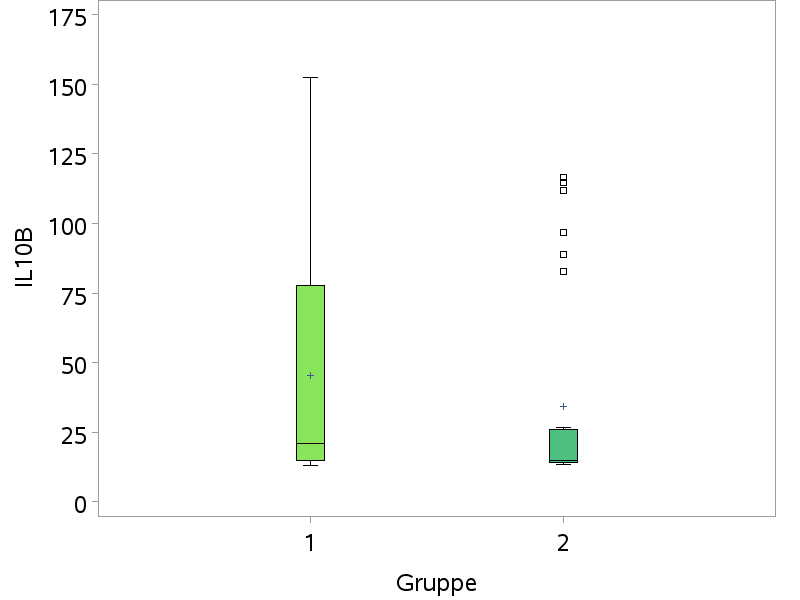## proc boxplot vaxis not working

Hello,

How can make proc boxplot actually use my vaxis tickmarks without changing the "ods graphics off;"? ("ods graphics off;" is needed in order to display the colors.)

The following code:

``````ods graphics off;
proc boxplot data=Simon4;
plot (IL10B)*Gruppe/ HEIGHT=4 HEIGHT=4 boxstyle=SCHEMATICID cboxes=black cboxfill=(MF_IL10) cvref = MF_IL10
vaxis=0 50 100 150;
where Gruppe in (1 2);
run;

``````

Delivers (instead of showing only the ticks at 0 50 100 150):## Re: proc boxplot vaxis not working

Hello @romangelzhaeuse,

I think SAS arbitrarily decides to deviate from the axis specification just because one or a few values in Gruppe 1 slightly exceed the maximum tick mark value of 150 (this behavior is really annoying), see warnings in the log:

```WARNING: For process variable IL10B the VAXIS= values specified do not cover the data range.
WARNING: Default scaling is substituted.```

A workaround is an axis specification that does "cover the data range" and to suppress the newly introduced tick mark values:

``axis1 order=(0 to 175 by 25) value=(t=2 '' t=4 '' t=6 '' t=8 '');``

(I think "0 to 200 by 50" would leave too much white space.)

Then specify vaxis=axis1 in the PLOT statement. More effort (probably involving the annotate feature) would be required to suppress the new tick marks, too.

Discussion stats